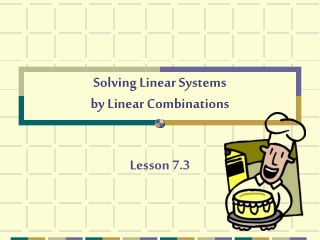DownloadDownload PresentationSolving Linear Systems by Linear Combinations

# Solving Linear Systems by Linear Combinations

Download Presentation## Solving Linear Systems by Linear Combinations

- - - - - - - - - - - - - - - - - - - - - - - - - - - E N D - - - - - - - - - - - - - - - - - - - - - - - - - - -
##### Presentation Transcript

1. Try adding this linear system together. { 4x + 3y = 1 2x – 3y = 1 Notice that the “y” terms in both equations are opposites of each other. If we add this system together, the “y” terms will cancel out and we can solve for “x”. 4x + 3y = 1 + 2x – 3y = 1 6x + 0 = 2 x = 1/3 Now that you solved for “x,” substitute it back into either equation and solve for “y.” 2(1/3) – 3y = 1 -3y = 1/3 y = -1/9 Solution (1/3, -1/9)

2. Important Steps: 1. Arrange equations with like terms in columns. 2. Study the xor y coefficients. Multiply one or both equations by an appropriate number to obtain new coefficients for x or y that are opposites. 3. Add equations. One of the variables will disappear. Solve for the remaining variable.

3. 4. Substitute the value in Step 3 into either of the original equations and solve for the other variable. You now have an ordered pair. 5. Check the solution in each original equation. If both are true, you have a solution.

4. 3x + 5y = 6 -4x + 2y = 5 What can we multiply by so that either the x or y value disappears? The top equation can be multiplied by 4 and the bottom equation by 3 to end up with 12x and –12x. When added together the x’s disappear. Solve for y. 12x + 20y = 24 (multiplied by 4) -12x + 6y = 15 (multiplied by 3) 26y = 39 (combined, now divide by 26) y = 3/2 (y value, plug in to find x value)

5. 3x + 5(3/2) = 6 (y value plugged in) 3x + 15/2 = 6 (now subtract 15/2) 3x = -3/2(now multiply by 1/3) x = - ½ Solution: (- 1/2, 3/2) Check: plug back into the original equations.

6. * Tip - When there are fractions in the equations, clear the fractions first! When to use which method????? Graph: to approximate a solution, check a solution or show visual. Substitution or combination: for exact solutions. Substitution: when one variable has 1 or –1 for the coefficient. Combination: when coefficients are larger.

7. Final Practice Problem… 8x + 2y = 16 5x – y = 28 Multiply the 2nd equation by 2 to cancel out the “y’s.” 8x + 2y = 16 10x – 2y = 56 8(4) + 2y = 16 2y = -16 y = -8 18x = 72 x = 4 Solution: (4, -8)